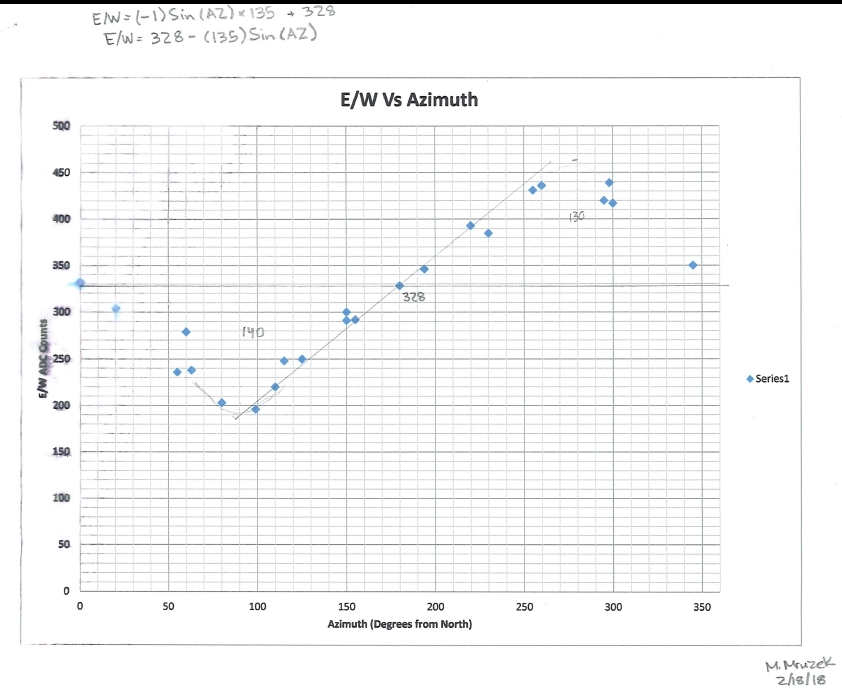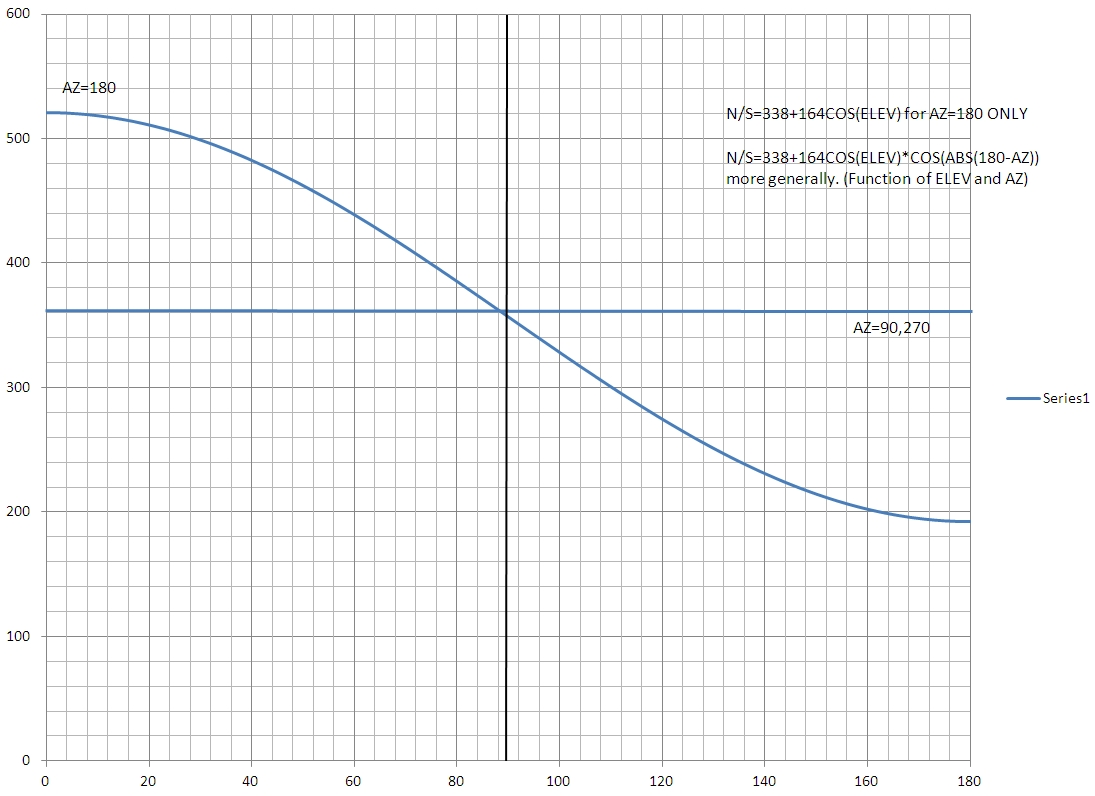# GPS Solar Tracker (3)

## MTM Scientific, Inc.

This series of webpages describes a GPS enabled solar tracker based upon the STMAX tilt-tilt platform design. The STMAX solar tracker controller is described in much more detail in our book "Build a Solar Tracker" and at http://www.mtmscientific.com/stmax.html.

The first webpage of this series described how to acquire time and position data from a GPS module. The second webpage described how to use the GPS time and position data to calculate the position of the sun in the sky. This webpage describes how to convert the aiming position in azimuth and elevation to ADC units on the STMAX tilt-tilt platform.

Calculating aim using a mathematical approach was found to be a very difficult problem. Eventually we had the idea to measure various random aiming directions and see if there is a predictable correlation to the tilt-tilt ADC counts for N/S and E/W. To our surprise there indeed was a very good correlation for both, as shown in Figures 1 and 2.Figure 1. East and South Correlation to ADC CountsFigure 2. North and South Correlation to ADC Counts

We were able to model both these correlations. The correlation formulas are written on the graphs. We modeled the East/West correlation as a sine wave. We modeled the North/South correlation as a simple line initially, however we later improved the correlation to be trigonometric using N/S = 338 + 164*[COS(ELEVATION)]*COS(ABS(180-AZ)). The trigonometric correlation is more accurate.  The concept of using ADC counts from the MEMS accelerometer is explained in our book.

Here are the improved results in graphical form:Figure 3. Improved North & South Correlation related to ADC Counts

We used these correlations to calculate aiming directions in units of ADC counts based upon the sun location calculated by the program described previously.

After using this correlation to track the sun we found that there was significant aiming error near the horizon. We did a more complete study of the problem and discovered the amplitude of the sinusoid varies according to the sine of the elevation. We show the correlation for 4 cases in Figure 4. (Results are plotted for 20, 45, 70 and 90 degrees of elevation). We see the sensitivity of the East & West aiming is reduced as the tracker moves closer to the horizon. This is to be expected based on the nature of the MEMS design.  The constant in the formula (338) is the ADC count when the tracker is level. The coefficient of the SIN calculation is 1/2 the same level ADC count. (Note: Both of these values vary slightly due to variance in the offset and sensitivity of the particular MEMS sensor. )Figure 4. Final North & South Correlation to ADC Counts